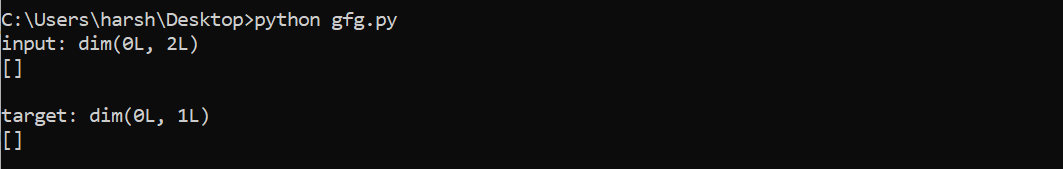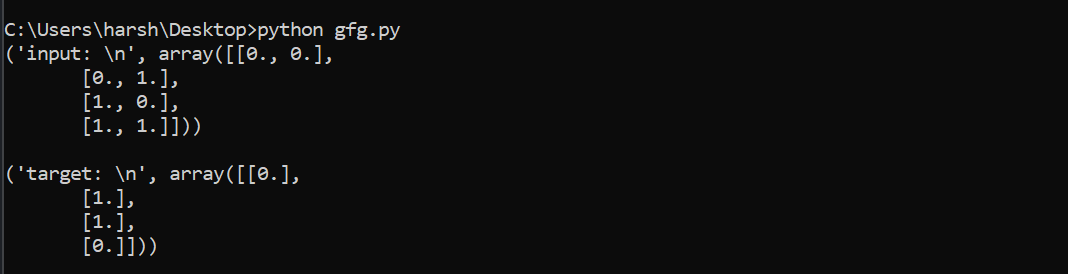# How to create a dataset using PyBrain?

• Last Updated : 07 Feb, 2022

In this article, we are going to see how to create a dataset using PyBrain.

## Dataset

Datasets are the data that are specifically given to test, validate and train on networks. Instead of troubling with arrays, PyBrain provides us with a more flexible data structure using which handling data can be quite easy.  A dataset can be displayed as a collection of named 2d-arrays. In machine learning, to handle each task it has special datasets.

## PyBrain

It is an acronym for Python-Based Reinforcement Learning, Artificial Intelligence. In Python, it is a modular Machine Learning Library. It provides flexible algorithms for doing machine learning tasks. It also provides a good environment to test algorithms. This article focuses on creating a dataset using PyBrain.

## Creating a dataset using PyBrain

To create a dataset using PyBrain one is required to use the pybrain.datasets (a dataset package of PyBrain). This dataset package offers the support of dataset classes. For example, it provides support for SequentialDataset, SupervisedDataset, ClassificationDataSet. The dataset that is to be used relies on the machine learning task that the programmer wants to implement. In this example, we are going to use SupervisedDataset. A SupervisedDataset dataset has the following syntax,

Syntax: SupervisedDataSet(input, target)

Where,

• input: A data structure (for example, 2-d array)
• target: The output

Example:

In this example, the input has a size equal to 2 and the target has a size equal to 1.

## Python3

 `# Python program to create a dataset ` `# using PyBrain ` ` `  `# Importing SupervisedDataSet from  ` `# pybrain.datasets ` `from` `pybrain.datasets ``import` `SupervisedDataSet ` ` `  `# Creating SupervisedDataSet ` `supervised_dataset ``=` `SupervisedDataSet(``2``, ``1``) ` ` `  `# Print ` `print``(dataSet) `

Output:In this part, we will discuss how we can add sample data to our data set.

Example:

In this example, we are creating an XOR truth table. The input passed is like a two-dimensional array and the output we get is 1. The input serves the purpose of size and the target acts as the output (equal is 1). So the inputs that are passed to our dataset are 2,1.

## Python3

 `# Python program to create a dataset  ` `# using PyBrain ` ` `  `# Importing supervised dataset from  ` `# pybrain.datasets ` `from` `pybrain.datasets ``import` `SupervisedDataSet ` ` `  `# Creating dataSet ` `supervised_dataset ``=` `SupervisedDataSet(``2``, ``1``) ` ` `  `# xor table ` `xor_table ``=` `[ ` `    ``[(``0``, ``0``), (``0``,)], ` `    ``[(``0``, ``1``), (``1``,)], ` `    ``[(``1``, ``0``), (``1``,)], ` `    ``[(``1``, ``1``), (``0``,)], ` `] ` ` `  `# Adding sample from xor_table into  ` `# supervised_dataset ` `for` `input``, target ``in` `xor_table: ` `    ``supervised_dataset.addSample(``input``, target) ` ` `  ` `  `# Printing the input ` `print``(``"input: \n"``, supervised_dataset[``'input'``]) ` ` `  `print``("") ` ` `  `# Printing the target ` `print``(``"target: \n"``, supervised_dataset[``'target'``]) `

Output:My Personal Notes arrow_drop_up
Recommended Articles
Page :